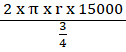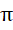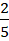# CAT 2019 Question Paper | Quants Slot 1

###### CAT Previous Year Paper | CAT Quants Questions | Question 32

Here is a classic mensuration question that you can easily answer if you had practiced CAT previous year paper question paper. It is about wheel revolutions and questions of this type have appeared several times in the past. A straightforward application of the formulas and some data manipulation should give the answer. Try cracking this one. If you find it hard, revisit the basics as part of your CAT Preparation journey.

Question 32 : The wheels of bicycles A and B have radii 30 cm and 40 cm, respectively. While traveling a certain distance, each wheel of A required 5000 more revolutions than each wheel of B. If bicycle B traveled this distance in 45 minutes, then its speed, in km per hour, was

1. 18π
2. 16π
3. 12π
4. 14π

## Best CAT Coaching in Chennai

#### CAT Coaching in Chennai - CAT 2022Limited Seats Available - Register Now!Circumference of A and B are in the ratio 3: 4
So, Ratio of Distance travelled in one revolution by A and B = 3: 4
Since they travel the same distance,
Ratio of number of revolutions of A and B = 4: 3 ----- (1)
We know that each wheel of A requires 5000 more revolutions than B
So, the Ratio of number of revolutions of A and B = (n + 5000): n ------(2)
So, comparing (1) and (2)
Number of revolutions of A and B are 20000 and 15000 respectively
So, we know Bike B does 15000 revolutions in 45 minutes
Distance travelled = 2 x π x r x Number of revolutions
Speed of Bike B =mph
Speed of Bike B = 2 xxx 5 x 4 Kmph
Speed of Bike B = 16Kmph

The question is "The wheels of bicycles A and B have radii 30 cm and 40 cm, respectively. While traveling a certain distance, each wheel of A required 5000 more revolutions than each wheel of B. If bicycle B traveled this distance in 45 minutes, then its speed, in km per hour, was"

##### Hence, the answer is 16π

Choice B is the correct answer.

###### CAT Coaching in ChennaiCAT 2023

Classroom Batches Starting Now! @Gopalapuram

###### Best CAT Coaching in Chennai Introductory offer of 5000/-

Attend a Demo Class

##### Where is 2IIM located?

2IIM Online CAT Coaching
A Fermat Education Initiative,
58/16, Indira Gandhi Street,
Kaveri Rangan Nagar, Saligramam, Chennai 600 093

##### How to reach 2IIM?

Mobile: (91) 99626 48484 / 94459 38484
WhatsApp: WhatsApp Now
Email: info@2iim.com